One litre of gas A and two litres of gas B, both having the same temperature 100$°$C and the same pressure 2.5 bar will have the ratio of kinetic energies of their molecules as:

(1) 1:1       (2) 1:2       (3) 1:4         (4) 4:1

Concept Questions :-

Kinetic energy of gas
High Yielding Test Series + Question Bank - NEET 2020

Difficulty Level:

On 0$°$C, the pressure measured by the barometer is 760 mm.  What will be pressure at 100$°$C?                                  [AFMC 2002]

(1) 760 mm        (2) 730 mm          (3) 780 mm           (4) None of these

Concept Questions :-

Ideal gas
High Yielding Test Series + Question Bank - NEET 2020

Difficulty Level:

By what percentage, should the pressure of a given mass of gas be increased, so as to decrease its volume by 10% at a constant temperature?

(1) 5%      (2) 7.2 %         (3) 12.5%          (4) 11.1%

Concept Questions :-

Ideal gas
High Yielding Test Series + Question Bank - NEET 2020

Difficulty Level:

In the adjacent V-T diagram what is the relation between  ?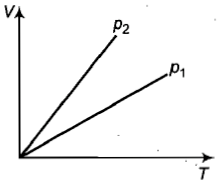(a) ${P}_{2}={P}_{1}$                         (b) ${P}_{2}>{P}_{1}$

(c) ${P}_{2}<{P}_{1}$                         (d) Cannot be predicated

Concept Questions :-

Ideal gas
High Yielding Test Series + Question Bank - NEET 2020

Difficulty Level:

The ratio $\frac{{C}_{p}}{{C}_{V}}=\gamma$ for a gas.  Its molecular weight is M. Its specification heat capacity at constant pressure is

(1) $\frac{R}{\gamma -1}$                (2) $\frac{\gamma R}{\gamma -1}$

(3) $\frac{\gamma R}{M\left(\gamma -1\right)}$         (4) $\frac{\gamma RM}{\left(\gamma -1\right)}$

Concept Questions :-

Specific heat
High Yielding Test Series + Question Bank - NEET 2020

Difficulty Level:

Which one of the following graph is correct at constant pressure

1.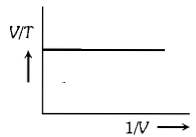2.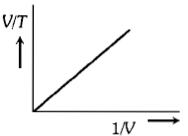3.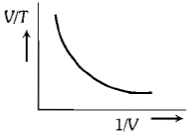4.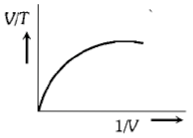Concept Questions :-

Ideal gas
High Yielding Test Series + Question Bank - NEET 2020

Difficulty Level:

The root-mean-square velocity of the molecules in a sample of helium is $\frac{5}{7}th$ of that of the molecules in a sample of hydrogen.  If the temperature of the hydrogen gas is 0$°$C, that of the helium sample is about:

(1) 0$°$C        (2) 4K         (3) 273$°$C         (4)100$°$C

Concept Questions :-

Types of velocity
High Yielding Test Series + Question Bank - NEET 2020

Difficulty Level:

Four molecules have speeds 2 km/sec, 3 km/sec, 4 km/sec and 5 km/sec.  The root mean square speed of these molecules (km/sec) is:

(1) $\sqrt{\frac{54}{4}}$          (2) $\sqrt{\frac{54}{2}}$          (3) 3.5            (4) 3$\sqrt{3}$

Concept Questions :-

Types of velocity
High Yielding Test Series + Question Bank - NEET 2020

Difficulty Level:

How many degrees of freedom the gas molecules have if, under STP, the gas density  and the velocity of sound propagation in it is 330 m${s}^{-1}$?

(1) 3       (2) 5           (3) 7              (4) 8

Concept Questions :-

Specific heat
High Yielding Test Series + Question Bank - NEET 2020

Difficulty Level:

The kinetic energy of one gram molecule of a gas at normal temperature and pressure is: (R = 8.31 J/mol-K)

[DPMT 1997; Pb. PMT 1997, 2000, 03; AFMC 1998; MH CET 1999]

(1) 0.56                (2)

(3)                   (4)

Concept Questions :-

Kinetic energy of gas